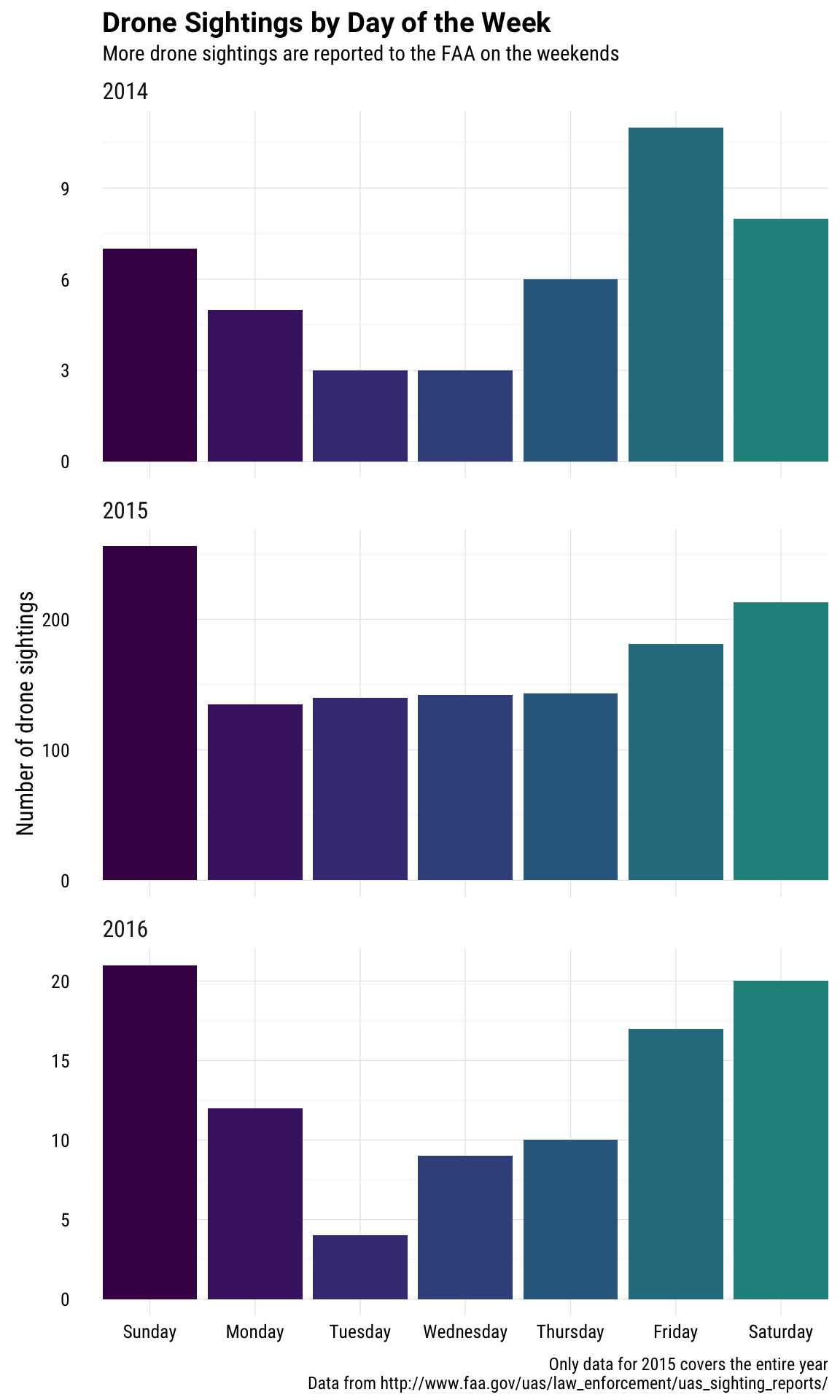Bob Rudis has a new weekly visualization challenge, and I am always up for practicing my plotting and visualization skills! The first challenge is to visualize a data set of unmanned aircraft (i.e. drone) sightings reported to the FAA. There have been some amazing submissions in this first challenge already (COUGH ANDREW HEISS COUGH), most of them centered on mapping the drone sightings, but I have been thinking about how these sightings are occurring/being reported in time vs. in space. Let’s explore how these drone sightings are distributed over the days of the week.

First, let’s download the two Excel files (in exactly the same way that Bob did in his example for the challenge). The two Excel files each contain drone sightings for different time periods.

library(readxl)
library(dplyr)
library(lubridate)
library(ggplot2)
library(viridis)

URL1 <- "http://www.faa.gov/uas/media/UAS_Sightings_report_21Aug-31Jan.xlsx"
URL2 <- "http://www.faa.gov/uas/media/UASEventsNov2014-Aug2015.xls"

fil1 <- basename(URL1)
fil2 <- basename(URL2)

xl2 <- read_excel(fil2)
## DEFINEDNAME: 00 00 00 06 0b 00 00 00 01 00 00 00 00 00 00 6f 77 73 73 76 72 3b 00 00 00 00 fc 02 00 00 05 00
## DEFINEDNAME: 00 00 00 06 0b 00 00 00 01 00 00 00 00 00 00 6f 77 73 73 76 72 3b 00 00 00 00 fc 02 00 00 05 00
## DEFINEDNAME: 00 00 00 06 0b 00 00 00 01 00 00 00 00 00 00 6f 77 73 73 76 72 3b 00 00 00 00 fc 02 00 00 05 00
## DEFINEDNAME: 00 00 00 06 0b 00 00 00 01 00 00 00 00 00 00 6f 77 73 73 76 72 3b 00 00 00 00 fc 02 00 00 05 00

Next, let’s bind the two data frames, keeping the timestamp, city, and state for each drone sighting. I am not keeping the report narrative, which certainly would be interesting to explore. There was 1 row with NA entries at this point that needs removing. Then let’s add a column with the year for each drone sighting. As Bob shows in his example visualization for this challenge, the sightings data begin in 2014, peak at high levels in 2015, and then taper off into 2016. This data set only covers a few weeks of 2014 and 2016 each. I’ve used the lubridate package to handle dates here, because it is so handy and lovely to use.

drones <- setNames(bind_rows(xl2[,1:3], xl1[,c(1,3,4)]),
c("timestamp", "city", "state"))
drones <- drones[!is.na(wday(drones$timestamp)),] drones <- drones %>% mutate(year = year(timestamp)) Now let’s plot. I’m choosing here to make a bar plot with an aesthetic where x = the weekday function from the lubridate package. I chose to facet on the year; there is not much data for 2014 or 2016, but when a distribution is so strongly peaked like this one (so many of the drone sightings came from late 2015), then the aggregate distribution across weekdays would be dominated by the behavior from late 2015. I want to see what the weekday distribution is like for more than just when drone sightings were at their peak. Let’s see! ggplot(data = drones, aes(x = wday(timestamp, label = TRUE, abbr = FALSE), fill = wday(timestamp, label = TRUE, abbr = FALSE))) + geom_bar() + theme_minimal(base_family = "RobotoCondensed-Regular", base_size = 12) + facet_wrap(~year, ncol = 1, scales = "free_y") + labs(title = "Drone Sightings by Day of the Week", subtitle = "More drone sightings are reported to the FAA on the weekends", y = "Number of drone sightings", caption = "Only data for 2015 covers the entire year\nData from http://www.faa.gov/uas/law_enforcement/uas_sighting_reports/") + scale_fill_viridis(end = 0.5, discrete=TRUE) + theme(plot.title=element_text(family="Roboto-Bold")) + theme(strip.text=element_text(size=12)) + theme(strip.text=element_text(hjust=0)) + theme(axis.text.x=element_text(margin=margin(t=-10))) + theme(plot.caption=element_text(margin=margin(t=10))) + theme(plot.caption=element_text(size=9)) + scale_x_discrete(expand=c(0,0)) + theme(legend.position = "none") + theme(axis.title.x=element_blank())Drone sightings are reported to the FAA at a higher level on weekend days than on weekdays. That is true across all the years in this data set, although it is good to keep in mind with this plot that the numbers are much higher for 2015 and that only 2015 has data that cover the entire year. This phenomenon of weekend drones fits in pretty well with the results of other challenge participants in that these drones seem to be largely belonging to hobbyists. If you are looking at those plots and wondering why 2015 looks so flat for Monday through Thursday while 2014 and 2016 do not, that is just due to random sampling. Let’s do a chi-squared test to confirm that. First, let’s look at the distribution for 2015. table(wday(drones[drones$year == 2015,]$timestamp, label = TRUE))[2:5] ## ## Mon Tues Wed Thurs ## 135 140 142 143 So even, right? What does the chi-squared test tell us about this distribution? Can we reject the null hypothesis that drone sightings occur at the same rate Monday through Thursday in 2015? chisq.test(table(wday(drones[drones$year == 2015,]$timestamp, label = TRUE))[2:5]) ## ## Chi-squared test for given probabilities ## ## data: table(wday(drones[drones$year == 2015, ]$timestamp, label = TRUE))[2:5] ## X-squared = 0.27143, df = 3, p-value = 0.9653 No, we cannot. This distribution is consistent with drone sightings occurring at the same rate Monday through Thursday. It turns out that this is true for the smaller samples in 2014 and 2016, though! chisq.test(table(wday(drones[drones$year == 2014,]$timestamp, label = TRUE))[2:5]) ## ## Chi-squared test for given probabilities ## ## data: table(wday(drones[drones$year == 2014, ]$timestamp, label = TRUE))[2:5] ## X-squared = 1.5882, df = 3, p-value = 0.6621 chisq.test(table(wday(drones[drones$year == 2016,]$timestamp, label = TRUE))[2:5]) ## ## Chi-squared test for given probabilities ## ## data: table(wday(drones[drones$year == 2016, ]$timestamp, label = TRUE))[2:5] ## X-squared = 3.9714, df = 3, p-value = 0.2646 In case you’re wondering, your eyes do not deceive you and this distribution is not consistent with being uniform across ALL the days of the week. chisq.test(table(wday(drones$timestamp, label = TRUE)))
##
##  Chi-squared test for given probabilities
##
## data:  table(wday(drones\$timestamp, label = TRUE))
## X-squared = 90.03, df = 6, p-value < 2.2e-16

Watch out for drones next weekend!